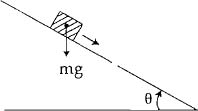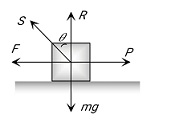Q

# Give answer! A plank with a box on it at one end is gradually raised about the other end. As the angle of inclination with the horizontal reaches 30°, the box starts to slip and slides 4.0 m down the plank in 4.0 s. The coefficients of static and

A plank with a box on it at one end is gradually raised about the other end. As the angle of inclination with the horizontal reaches 30°, the box starts to slip and slides 4.0 m down the plank in 4.0 s. The coefficients of static and kinetic friction between the box and the plank will be, respectively:• Option 1)

0.6 and 0.5

• Option 2)

0.5 and 0.6

• Option 3)

0.4 and 0.3

• Option 4)

0.6 and 0.6

1003 Views

As we learnt in

Angle of Friction -Angle  is called angle of friction.

R = Reaction,

Force of limiting friction

- wherein

static friction coefficient

Angle made by resultant of limiting friction and R with the normal reaction.

Correct option is 1.

Option 1)

0.6 and 0.5

Correct

Option 2)

0.5 and 0.6

Incorrect

Option 3)

0.4 and 0.3

Incorrect

Option 4)

0.6 and 0.6

Incorrect

Exams
Articles
Questions